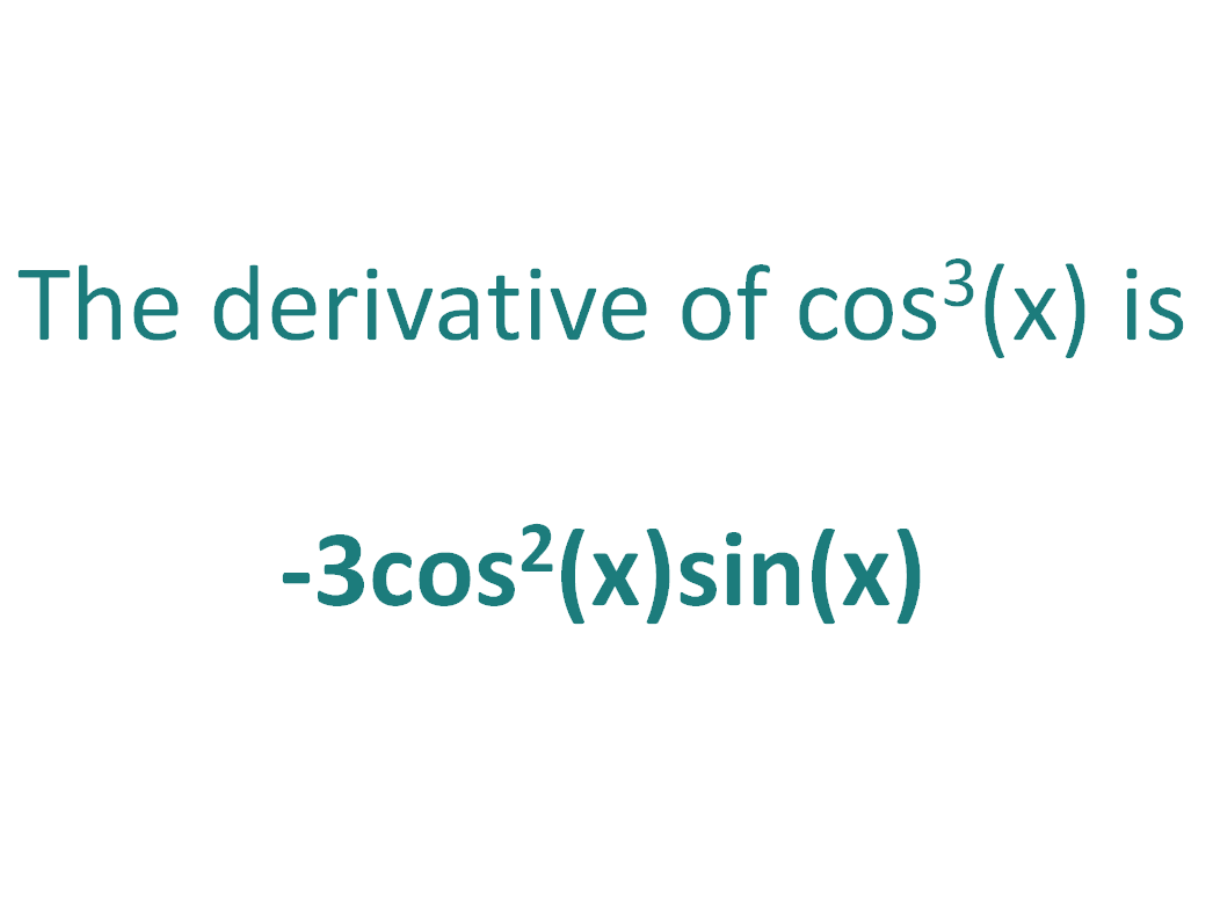# The Derivative of cos^3xThe derivative of cos^3x is -3cos2(x)sin(x)

## How to calculate the derivative of cos^3x

Note that in this post we will be looking at differentiating cos3(x) which is not the same as differentiating cos(3x). Here is our post dealing with how to differentiate cos(3x).

The chain rule is useful for finding the derivative of a function which could have been differentiated had it been in x, but it is in the form of another expression which could also be differentiated if it stood on its own.

In this case:

• We know how to differentiate cos(x) (the answer is -sin(x))
• We know how to differentiate x3 (the answer is 3x2)

This means the chain rule will allow us to perform the differentiation of the expression cos3(x).

#### Using the chain rule to find the derivative of cos^3x

Although the expression cos3x contains no parenthesis, we can still view it as a composite function (a function of a function).

We can write cos3x as (cos(x))3.

Now the function is in the form of x3, except it does not have x as the base, instead it has another function of x (cos(x)) as the base.

Let’s call the function of the base g(x), which means:

g(x) = cos(x)

From this it follows that:

cos(x)3 = g(x)3

So if the function f(x) = x3 and the function g(x) = cos(x), then the function (cos(x))3 can be written as a composite function.

f(x) = x3

f(g(x)) = g(x)3 (but g(x) = cos(x))

f(g(x)) = (cos(x))3

Let’s define this composite function as F(x):

F(x) = f(g(x)) = (cos(x))3

We can find the derivative of cos^3x (F'(x)) by making use of the chain rule.

The Chain Rule:
For two differentiable functions f(x) and g(x)

If F(x) = f(g(x))

Then the derivative of F(x) is F'(x) = f’(g(x)).g’(x)

Now we can just plug f(x) and g(x) into the chain rule.

How to find the derivative of cos^3x using the Chain Rule:

Using the chain rule, the derivative of cos^3x is -3cos2(x)sin(x)

Finally, just a note on syntax and notation: cos^3x is sometimes written in the forms below (with the derivative as per the calculations above). Just be aware that not all of the forms below are mathematically correct.

## The Second Derivative Of cos^3x

To calculate the second derivative of a function, you just differentiate the first derivative.

From above, we found that the first derivative of cos^3x = -3cos2(x)sin(x). So to find the second derivative of cos^3x, we just need to differentiate -3cos2(x)sin(x).

We can use the product rule and trig identities to find the derivative of -3cos2(x)sin(x) and we get an answer of -3cos3(x) + 3sin(x)sin(2x)

The second derivative of cos^3x is -3cos3(x) + 3sin(x)sin(2x)